# US20110037498A1 - Vlsi layouts of fully connected generalized networks - Google Patents

## Info

Publication number
US20110037498A1
US20110037498A1 US12/601,275 US60127508A US2011037498A1 US 20110037498 A1 US20110037498 A1 US 20110037498A1 US 60127508 A US60127508 A US 60127508A US 2011037498 A1 US2011037498 A1 US 2011037498A1
Authority
US
United States
Prior art keywords
integrated circuit
stage
switches
sub
Prior art date
Legal status (The legal status is an assumption and is not a legal conclusion. Google has not performed a legal analysis and makes no representation as to the accuracy of the status listed.)
Granted
Application number
US12/601,275
Other versions
US8269523B2 (en
Inventor
Venkat Konda
Current Assignee (The listed assignees may be inaccurate. Google has not performed a legal analysis and makes no representation or warranty as to the accuracy of the list.)
Individual
Original Assignee
Individual
Priority date (The priority date is an assumption and is not a legal conclusion. Google has not performed a legal analysis and makes no representation as to the accuracy of the date listed.)
Filing date
Publication date
Family has litigation
Application filed by Individual filed Critical Individual
Priority to US12/601,275 priority Critical patent/US8269523B2/en
Publication of US20110037498A1 publication Critical patent/US20110037498A1/en
Application granted granted Critical
Publication of US8269523B2 publication Critical patent/US8269523B2/en
Assigned to Konda Technologies Inc. reassignment Konda Technologies Inc. ASSIGNMENT OF ASSIGNORS INTEREST (SEE DOCUMENT FOR DETAILS). Assignors: KONDA, VENKAT
Assigned to KONDA, VENKAT reassignment KONDA, VENKAT ASSIGNMENT OF ASSIGNORS INTEREST (SEE DOCUMENT FOR DETAILS). Assignors: Konda Technologies Inc.
Active legal-status Critical Current

## Images

•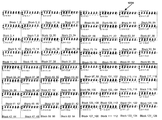•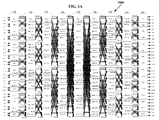•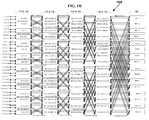•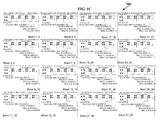••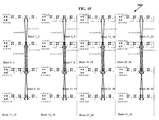•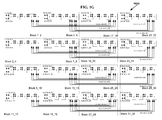••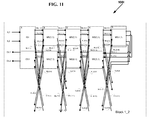•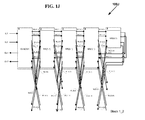•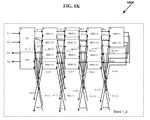•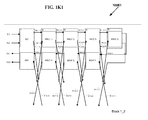•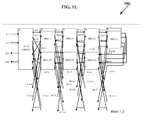•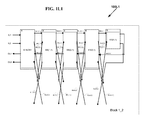•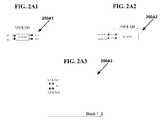•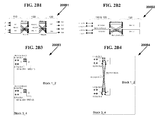•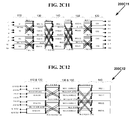••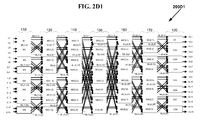•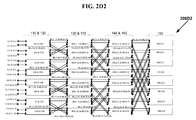•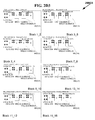•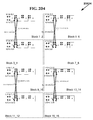•••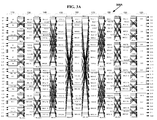•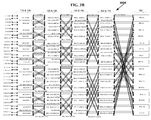•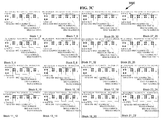•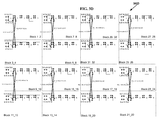•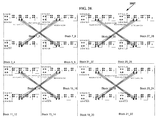••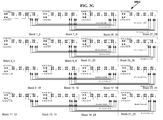•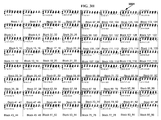•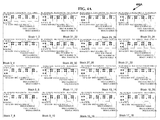•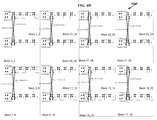•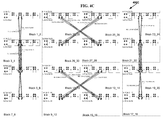•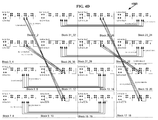•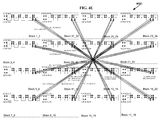•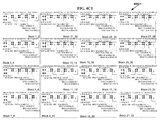•## Classifications

• GPHYSICS
• G06COMPUTING; CALCULATING OR COUNTING
• G06FELECTRIC DIGITAL DATA PROCESSING
• G06F30/30Circuit design
• G06F30/39Circuit design at the physical level
• G06F30/394Routing

## Abstract

In accordance with the invention, VLSI layouts of generalized multi-stage networks for broadcast, unicast and multicast connections are presented using only horizontal and vertical links. The VLSI layouts employ shuffle exchange links where outlet links of cross links from switches in a stage in one sub-integrated circuit block are connected to inlet links of switches in the succeeding stage in another sub-integrated circuit block so that said cross links are either vertical links or horizontal and vice versa. In one embodiment the sub-integrated circuit blocks are arranged in a hypercube arrangement in a two-dimensional plane. The VLSI layouts exploit the benefits of significantly lower cross points, lower signal latency, lower power and full connectivity with significantly fast compilation.
The VLSI layouts presented are applicable to generalized multi-stage networks V(N1, N2, d, s), generalized folded multi-stage networks Vfold(N1, N2, d, s), generalized butterfly fat tree networks Vbft(N1, N2, d, s), generalized multi-link multi-stage networks Vmlink(N1, N2, d, s), generalized folded multi-link multi-stage networks Vfold-mlink(N1, N2, d, s), generalized multi-link butterfly fat tree networks Vmlink-bft(N1, N2, d, s), and generalized hypercube networks Vhcube(N1, N2, d, s) for s=1, 2, 3 or any number in general. The embodiments of VLSI layouts are useful in wide target applications such as FPGAs, CPLDs, pSoCs, ASIC placement and route tools, networking applications, parallel & distributed computing, and reconfigurable computing.

## Description

CROSS REFERENCE TO RELATED APPLICATIONS
• This application is related to and claims priority of the PCT Application Serial No. PCT/US08/64605 entitled “VLSI LAYOUTS OF FULLY CONNECTED GENERALIZED NETWORKS” by Venkat Konda assigned to the same assignee as the current application, filed May 22, 2008, and the U.S. Provisional Patent Application Ser. No. 60/940,394 entitled “VLSI LAYOUTS OF FULLY CONNECTED GENERALIZED NETWORKS” by Venkat Konda assigned to the same assignee as the current application, filed May 25, 2007.
• This application is related to and incorporates by reference in its entirety the U.S. application Ser. No. 12/530,207 entitled “FULLY CONNECTED GENERALIZED MULTI-STAGE NETWORKS” by Venkat Konda assigned to the same assignee as the current application, filed Sep. 6, 2009, the PCT Application Serial No. PCT/US08/56064 entitled “FULLY CONNECTED GENERALIZED MULTI-STAGE NETWORKS” by Venkat Konda assigned to the same assignee as the current application, filed Mar. 6, 2008, the U.S. Provisional Patent Application Ser. No. 60/905,526 entitled “LARGE SCALE CROSSPOINT REDUCTION WITH NONBLOCKING UNICAST & MULTICAST IN ARBITRARILY LARGE MULTI-STAGE NETWORKS” by Venkat Konda assigned to the same assignee as the current application, filed Mar. 6, 2007, and the U.S. Provisional Patent Application Ser. No. 60/940,383 entitled “FULLY CONNECTED GENERALIZED MULTI-STAGE NETWORKS” by Venkat Konda assigned to the same assignee as the current application, filed May 25, 2007.
• This application is related to and incorporates by reference in its entirety the US Patent Application Docket No. V-0038US entitled “FULLY CONNECTED GENERALIZED BUTTERFLY FAT TREE NETWORKS” by Venkat Konda assigned to the same assignee as the current application filed concurrently, the PCT Application Serial No. PCT/US08/64603 entitled “FULLY CONNECTED GENERALIZED BUTTERFLY FAT TREE NETWORKS” by Venkat Konda assigned to the same assignee as the current application, filed May 22, 2008, the U.S. Provisional Patent Application Ser. No. 60/940, 387 entitled “FULLY CONNECTED GENERALIZED BUTTERFLY FAT TREE NETWORKS” by Venkat Konda assigned to the same assignee as the current application, filed May 25, 2007, and the U.S. Provisional Patent Application Ser. No. 60/940, 390 entitled “FULLY CONNECTED GENERALIZED MULTI-LINK BUTTERFLY FAT TREE NETWORKS” by Venkat Konda assigned to the same assignee as the current application, filed May 25, 2007.
• This application is related to and incorporates by reference in its entirety the US Patent Application Docket No. V-0039US entitled “FULLY CONNECTED GENERALIZED MULTI-LINK MULTI-STAGE NETWORKS” by Venkat Konda assigned to the same assignee as the current application filed concurrently, the PCT Application Serial No. PCT/US08/64604 entitled “FULLY CONNECTED GENERALIZED MULTI-LINK MULTI-STAGE NETWORKS” by Venkat Konda assigned to the same assignee as the current application, filed May 22, 2008, the U.S. Provisional Patent Application Ser. No. 60/940, 389 entitled “FULLY CONNECTED GENERALIZED REARRANGEABLY NONBLOCKING MULTI-LINK MULTI-STAGE NETWORKS” by Venkat Konda assigned to the same assignee as the current application, filed May 25, 2007, the U.S. Provisional Patent Application Ser. No. 60/940, 391 entitled “FULLY CONNECTED GENERALIZED FOLDED MULTI-STAGE NETWORKS” by Venkat Konda assigned to the same assignee as the current application, filed May 25, 2007 and the U.S. Provisional Patent Application Ser. No. 60/940,392 entitled “FULLY CONNECTED GENERALIZED STRICTLY NONBLOCKING MULTI-LINK MULTI-STAGE NETWORKS” by Venkat Konda assigned to the same assignee as the current application, filed May 25, 2007.
• This application is related to and incorporates by reference in its entirety the U.S. Provisional Patent Application Ser. No. 61/252, 603 entitled “VLSI LAYOUTS OF FULLY CONNECTED NETWORKS WITH LOCALITY EXPLOITATION” by Venkat Konda assigned to the same assignee as the current application, filed Oct. 16, 2009.
• This application is related to and incorporates by reference in its entirety the U.S. Provisional Patent Application Ser. No. 61/252, 609 entitled “VLSI LAYOUTS OF FULLY CONNECTED GENERALIZED AND PYRAMID NETWORKS” by Venkat Konda assigned to the same assignee as the current application, filed Oct. 16, 2009.
• BACKGROUND OF INVENTION
• Multi-stage interconnection networks such as Benes networks and butterfly fat tree networks are widely useful in telecommunications, parallel and distributed computing. However VLSI layouts, known in the prior art, of these interconnection networks in an integrated circuit are inefficient and complicated.
• Other multi-stage interconnection networks including butterfly fat tree networks, Banyan networks, Batcher-Banyan networks, Baseline networks, Delta networks, Omega networks and Flip networks have been widely studied particularly for self routing packet switching applications. Also Benes Networks with radix of two have been widely studied and it is known that Benes Networks of radix two are shown to be built with back to back baseline networks which are rearrangeably nonblocking for unicast connections.
• The most commonly used VLSI layout in an integrated circuit is based on a two-dimensional grid model comprising only horizontal and vertical tracks. An intuitive interconnection network that utilizes two-dimensional grid model is 2D Mesh Network and its variations such as segmented mesh networks. Hence routing networks used in VLSI layouts are typically 2D mesh networks and its variations. However Mesh Networks require large scale cross points typically with a growth rate of O(N2) where N is the number of computing elements, ports, or logic elements depending on the application.
• Multi-stage interconnection with a growth rate of O(N×log N) requires significantly small number of cross points. U.S. Pat. No. 6,185,220 entitled “Grid Layouts of Switching and Sorting Networks” granted to Muthukrishnan et al. describes a VLSI layout using existing VLSI grid model for Benes and Butterfly networks. U.S. Pat. No. 6,940,308 entitled “Interconnection Network for a Field Programmable Gate Array” granted to Wong describes a VLSI layout where switches belonging to lower stage of Benes Network are layed out close to the logic cells and switches belonging to higher stages are layed out towards the center of the layout.
• Due to the inefficient and in some cases impractical VLSI layout of Benes and butterfly fat tree networks on a semiconductor chip, today mesh networks and segmented mesh networks are widely used in the practical applications such as field programmable gate arrays (FPGAs), programmable logic devices (PLDs), and parallel computing interconnects. The prior art VLSI layouts of Benes and butterfly fat tree networks and VLSI layouts of mesh networks and segmented mesh networks require large area to implement the switches on the chip, large number of wires, longer wires, with increased power consumption, increased latency of the signals which effect the maximum clock speed of operation. Some networks may not even be implemented practically on a chip due to the lack of efficient layouts.
• SUMMARY OF INVENTION
• When large scale sub-integrated circuit blocks with inlet and outlet links are layed out in an integrated circuit device in a two-dimensional grid arrangement, (for example in an FPGA where the sub-integrated circuit blocks are Lookup Tables) the most intuitive routing network is a network that uses horizontal and vertical links only (the most often used such a network is one of the variations of a 2D Mesh network). A direct embedding of a generalized multi-stage network on to a 2D Mesh network is neither simple nor efficient.
• In accordance with the invention, VLSI layouts of generalized multi-stage networks for broadcast, unicast and multicast connections are presented using only horizontal and vertical links The VLSI layouts employ shuffle exchange links where outlet links of cross links from switches in a stage in one sub-integrated circuit block are connected to inlet links of switches in the succeeding stage in another sub-integrated circuit block so that said cross links are either vertical links or horizontal and vice versa. In one embodiment the sub-integrated circuit blocks are arranged in a hypercube arrangement in a two-dimensional plane. The VLSI layouts exploit the benefits of significantly lower cross points, lower signal latency, lower power and full connectivity with significantly fast compilation.
• The VLSI layouts presented are applicable to generalized multi-stage networks V(N1, N2, d, s), generalized folded multi-stage networks Vfold(N1, N2, d, s), generalized butterfly fat tree networks Vbft(N1, N2, d, s), generalized multi-link multi-stage networks Vmlink(N1, N2, d, s), generalized folded multi-link multi-stage networks Vfold-mlink(N1, N2, d, s), generalized multi-link butterfly fat tree networks Vmlink-bft(N1, N2, d, s), and generalized hypercube networks Vhcube(N1, N2, d, s) for s=1, 2, 3 or any number in general. The embodiments of VLSI layouts are useful in wide target applications such as FPGAs, CPLDs, pSoCs, ASIC placement and route tools, networking applications, parallel & distributed computing, and reconfigurable computing.
• BRIEF DESCRIPTION OF DRAWINGS
• FIG. 1A is a diagram 100A of an exemplary symmetrical multi-link multi-stage network Vfold-mlink(N, d, s) having inverse Benes connection topology of nine stages with N=32, d=2 and s=2, strictly nonblocking network for unicast connections and rearrangeably nonblocking network for arbitrary fan-out multicast connections, in accordance with the invention.
• FIG. 1B is a diagram 100B of the equivalent symmetrical folded multi-link multi-stage network Vfold-mlink(N, d, s) of the network 100A shown in FIG. 1A, having inverse Benes connection topology of five stages with N=32, d=2 and s=2, strictly nonblocking network for unicast connections and rearrangeably nonblocking network for arbitrary fan-out multicast connections, in accordance with the invention.
• FIG. 1C is a diagram 100C layout of the network Vfold-mlink(N, d, s) shown in FIG. 1B, in one embodiment, illustrating the connection links belonging with in each block only.
• FIG. 1D is a diagram 100D layout of the network Vfold-mlink(N, d, s) shown in FIG. 1B, in one embodiment, illustrating the connection links ML(1,i) for i=[1, 64] and ML(8,i) for i=[1,64].
• FIG. 1E is a diagram 100E layout of the network Vfold-mlink(N, d, s) shown in FIG. 1B, in one embodiment, illustrating the connection links ML(2,i) for i=[1, 64] and ML(7,i) for i=[1,64].
• FIG. 1F is a diagram 100F layout of the network Vfold-mlink(N, d, s) shown in FIG. 1B, in one embodiment, illustrating the connection links ML(3,i) for i=[1, 64] and ML(6,i) for i=[1,64].
• FIG. 1G is a diagram 100G layout of the network Vfold-mlink(N, d, s) shown in FIG. 1B, in one embodiment, illustrating the connection links ML(4,i) for i=[1, 64] and ML(5,i) for i=[1,64].
• FIG. 1H is a diagram 100H layout of a network Vfold-mlink(N, d, s) where N=128, d=2, and s=2, in one embodiment, illustrating the connection links belonging with in each block only.
• FIG. 11 is a diagram 100I detailed connections of BLOCK 1_2 in the network layout 100C in one embodiment, illustrating the connection links going in and coming out when the layout 100C is implementing V(N, d, s) or Vfold(N, d, s).
• FIG. 1J is a diagram 100J detailed connections of BLOCK 1_2 in the network layout 100C in one embodiment, illustrating the connection links going in and coming out when the layout 100C is implementing V(N, d, s) or Vfold(N, d, s).
• FIG. 1K is a diagram 100K detailed connections of BLOCK 1_2 in the network layout 100C in one embodiment, illustrating the connection links going in and coming out when the layout 100C is implementing V(N, d, s) or Vfold(N, d, s).
• FIG. 1K1 is a diagram 100M1 detailed connections of BLOCK 1_2 in the network layout 100C in one embodiment, illustrating the connection links going in and coming out when the layout 100C is implementing V(N, d, s) or Vfold(N, d, s) for s=1.
• FIG. 1L is a diagram 100L detailed connections of BLOCK 1_2 in the network layout 100C in one embodiment, illustrating the connection links going in and coming out when the layout 100C is implementing V(N, d, s) or Vfold(N, d, s).
• FIG. 1L1 is a diagram 100L1 detailed connections of BLOCK 1_2 in the network layout 100C in one embodiment, illustrating the connection links going in and coming out when the layout 100C is implementing V(N, d, s) or Vfold(N, d, s) for s=1.
• FIG. 2A1 is a diagram 200A1 of an exemplary symmetrical multi-link multi-stage network Vfold-mlink(N, d, s) having inverse Benes connection topology of one stage with N=2, d=2 and s=2, strictly nonblocking network for unicast connections and rearrangeably nonblocking network for arbitrary fan-out multicast connections, in accordance with the invention. FIG. 2A2 is a diagram 200A2 of the equivalent symmetrical folded multi-link multi-stage network Vfold-mlink(N, d, s) of the network 200A1 shown in FIG. 2A1, having inverse Benes connection topology of one stage with N=2, d=2 and s=2, strictly nonblocking network for unicast connections and rearrangeably nonblocking network for arbitrary fan-out multicast connections, in accordance with the invention. FIG. 2A3 is a diagram 200A3 layout of the network Vfold-mlink(N, d, s) shown in FIG. 2A2, in one embodiment, illustrating all the connection links.
• FIG. 2B1 is a diagram 200B1 of an exemplary symmetrical multi-link multi-stage network Vfold-mlink(N, d, s) having inverse Benes connection topology of one stage with N=4, d=2 and s=2, strictly nonblocking network for unicast connections and rearrangeably nonblocking network for arbitrary fan-out multicast connections, in accordance with the invention. FIG. 2B2 is a diagram 200B2 of the equivalent symmetrical folded multi-link multi-stage network Vfold-mlink(N, d, s) of the network 200B1 shown in FIG. 2B1, having inverse Benes connection topology of one stage with N=4, d=2 and s=2, strictly nonblocking network for unicast connections and rearrangeably nonblocking network for arbitrary fan-out multicast connections, in accordance with the invention. FIG. 2B3 is a diagram 200B3 layout of the network Vfold-mlink(N, d, s) shown in FIG. 2B2, in one embodiment, illustrating the connection links belonging with in each block only. FIG. 2B4 is a diagram 200B4 layout of the network Vfold-mlink(N, d, s) shown in FIG. 2B2, in one embodiment, illustrating the connection links ML(1,i) for i=[1, 8] and ML(2,i) for i=[1,8].
• FIG. 2C11 is a diagram 200C11 of an exemplary symmetrical multi-link multi-stage network Vfold-mlink(N, d, s) having inverse Benes connection topology of one stage with N=8, d=2 and s=2, strictly nonblocking network for unicast connections and rearrangeably nonblocking network for arbitrary fan-out multicast connections, in accordance with the invention. FIG. 2C12 is a diagram 200C12 of the equivalent symmetrical folded multi-link multi-stage network Vfold-mlink(N, d, s) of the network 200C11 shown in FIG. 2C11, having inverse Benes connection topology of one stage with N=8, d=2 and s=2, strictly nonblocking network for unicast connections and rearrangeably nonblocking network for arbitrary fan-out multicast connections, in accordance with the invention.
• FIG. 2C21 is a diagram 200C21 layout of the network Vfold-mlink(N, d, s) shown in FIG. 2C12, in one embodiment, illustrating the connection links belonging with in each block only. FIG. 2C22 is a diagram 200C22 layout of the network Vfold-mlink(N, d, s) shown in FIG. 2C12, in one embodiment, illustrating the connection links ML(1,i) for i=[1, 16] and ML(4,i) for i=[1,16]. FIG. 2C23 is a diagram 200C23 layout of the network Vfold-mlink(N, d, s) shown in FIG. 2C12, in one embodiment, illustrating the connection links ML(2,i) for i=[1, 16] and ML(3,i) for i=[1,16].
• FIG. 2D1 is a diagram 200D1 of an exemplary symmetrical multi-link multi-stage network Vfold-mlink(N, d, s) having inverse Benes connection topology of one stage with N=16, d=2 and s=2, strictly nonblocking network for unicast connections and rearrangeably nonblocking network for arbitrary fan-out multicast connections, in accordance with the invention.
• FIG. 2D2 is a diagram 200D2 of the equivalent symmetrical folded multi-link multi-stage network Vfold-mlink(N, d, s) of the network 200D1 shown in FIG. 2D1, having inverse Benes connection topology of one stage with N=16, d=2 and s=2, strictly nonblocking network for unicast connections and rearrangeably nonblocking network for arbitrary fan-out multicast connections, in accordance with the invention.
• FIG. 2D3 is a diagram 200D3 layout of the network Vfold-mlink(N, d, s) shown in FIG. 2D2, in one embodiment, illustrating the connection links belonging with in each block only.
• FIG. 2D4 is a diagram 200D4 layout of the network Vfold-mlink(N, d, s) shown in FIG. 2D2, in one embodiment, illustrating the connection links ML(1,i) for i=[1, 32] and ML(6,i) for i=[1,32].
• FIG. 2D5 is a diagram 200D5 layout of the network Vfold-mlink(N, d, s) shown in FIG. 2D2, in one embodiment, illustrating the connection links ML(2,i) for i=[1, 32] and ML(5,i) for i=[1,32].
• FIG. 2D6 is a diagram 200D6 layout of the network Vfold-mlink(N, d, s) shown in FIG. 2D2, in one embodiment, illustrating the connection links ML(3,i) for i=[1, 32] and ML(4,i) for i=[1,32].
• FIG. 3A is a diagram 300A of an exemplary symmetrical multi-link multi-stage network Vhcube(N, d, s) having inverse Benes connection topology of nine stages with N=32, d=2 and s=2, strictly nonblocking network for unicast connections and rearrangeably nonblocking network for arbitrary fan-out multicast connections, in accordance with the invention.
• FIG. 3B is a diagram 300B of the equivalent symmetrical folded multi-link multi-stage network Vhcube(N, d, s) of the network 300A shown in FIG. 3A, having inverse Benes connection topology of five stages with N=32, d=2 and s=2, strictly nonblocking network for unicast connections and rearrangeably nonblocking network for arbitrary fan-out multicast connections, in accordance with the invention.
• FIG. 3C is a diagram 300C layout of the network Vhcube(N, d, s) shown in FIG. 3B, in one embodiment, illustrating the connection links belonging with in each block only.
• FIG. 3D is a diagram 100D layout of the network Vhcube(N, d, s) shown in FIG. 3B, in one embodiment, illustrating the connection links ML(1,i) for i=[1, 64] and ML(8,i) for i=[1,64].
• FIG. 3E is a diagram 300E layout of the network Vhcube(N, d, s) shown in FIG. 3B, in one embodiment, illustrating the connection links ML(2,i) for i=[1, 64] and ML(7,i) for i=[1,64].
• FIG. 3F is a diagram 300F layout of the network Vhcube(N, d, s) shown in FIG. 3B, in one embodiment, illustrating the connection links ML(3,i) for i=[1, 64] and ML(6,i) for i=[1,64].
• FIG. 3G is a diagram 300G layout of the network Vhcube(N, d, s) shown in FIG. 3B, in one embodiment, illustrating the connection links ML(4,i) for i=[1, 64] and ML(5,i) for i=[1,64].
• FIG. 3H is a diagram 300H layout of a network Vhcube(N, d, s) where N=128, d=2, and s=2, in one embodiment, illustrating the connection links belonging with in each block only.
• FIG. 4A is a diagram 400A layout of the network Vfold-mlink(N, d, s) shown in FIG. 1B, in one embodiment, illustrating the connection links belonging with in each block only.
• FIG. 4B is a diagram 400B layout of the network Vfold- mlink(N, d, s) shown in FIG. 1B, in one embodiment, illustrating the connection links ML(1,i) for i=[1, 64] and ML(8,i) for i=[1,64].
• FIG. 4C is a diagram 400C layout of the network Vfold-mlink(N, d, s) shown in FIG. 4C, in one embodiment, illustrating the connection links ML(2,i) for i=[1, 64] and ML(7,i) for i=[1,64].
• FIG. 4D is a diagram 400D layout of the network Vfold-mlink(N, d, s) shown in FIG. 4D, in one embodiment, illustrating the connection links ML(3,i) for i=[1, 64] and ML(6,i) for i=[1,64].
• FIG. 4E is a diagram 400E layout of the network Vfold-mlink(N, d, s) shown in FIG. 4E, in one embodiment, illustrating the connection links ML(4,i) for i=[1, 64] and ML(5,i) for i=[1,64].
• FIG. 4C1 is a diagram 400C1 layout of the network Vfold-mlink(N, d, s) shown in FIG. 1B, in one embodiment, illustrating the connection links belonging with in each block only.
• FIG. 5A1 is a diagram 500A1 of an exemplary prior art implementation of a two by two switch; FIG. 5A2 is a diagram 500A2 for programmable integrated circuit prior art implementation of the diagram 500A1 of FIG. 5A1; FIG. 5A3 is a diagram 500A3 for one-time programmable integrated circuit prior art implementation of the diagram 500A1 of FIG. 5A1; FIG. 5A4 is a diagram 500A4 for integrated circuit placement and route implementation of the diagram 500A1 of FIG. 5A1.
• DETAILED DESCRIPTION OF THE INVENTION
• The present invention is concerned with the VLSI layouts of arbitrarily large switching networks for broadcast, unicast and multicast connections. Particularly switching networks considered in the current invention include: generalized multi-stage networks V(N1, N2, d, s), generalized folded multi-stage networks Vfold(N1, N2, d, s), generalized butterfly fat tree networks Vbft(N1, N2, d, s), generalized multi-link multi-stage networks Vmlink(N1, N2, d, s), generalized folded multi-link multi-stage networks Vfold-mlink(N1, N2, d, s), generalized multi-link butterfly fat tree networks Vmlink-bft(N1, N2, d, s), and generalized hypercube networks Vhcube(N1, N2, d, s) for s=1, 2, 3 or any number in general.
• Efficient VLSI layout of networks on a semiconductor chip are very important and greatly influence many important design parameters such as the area taken up by the network on the chip, total number of wires, length of the wires, latency of the signals, capacitance and hence the maximum clock speed of operation. Some networks may not even be implemented practically on a chip due to the lack of efficient layouts. The different varieties of multi-stage networks described above have not been implemented previously on the semiconductor chips efficiently. For example in Field Programmable Gate Array (FPGA) designs, multi-stage networks described in the current invention have not been successfully implemented primarily due to the lack of efficient VLSI layouts. Current commercial FPGA products such as Xilinx Vertex, Altera's Stratix implement island-style architecture using mesh and segmented mesh routing interconnects using either full crossbars or sparse crossbars. These routing interconnects consume large silicon area for crosspoints, long wires, large signal propagation delay and hence consume lot of power.
• The current invention discloses the VLSI layouts of numerous types of multi-stage networks which are very efficient. Moreover they can be embedded on to mesh and segmented mesh routing interconnects of current commercial FPGA products. The VLSI layouts disclosed in the current invention are applicable to including the numerous generalized multi-stage networks disclosed in the following patent applications, filed concurrently:
• 1) Strictly and rearrangeably nonblocking for arbitrary fan-out multicast and unicast for generalized multi-stage networks V(N1, N2, d, s) with numerous connection topologies and the scheduling methods are described in detail in the PCT Application Serial No. PCT/US08/56064 that is incorporated by reference above.
• 2) Strictly and rearrangeably nonblocking for arbitrary fan-out multicast and unicast for generalized butterfly fat tree networks Vbft(N1, N2, d, s) with numerous connection topologies and the scheduling methods are described in detail in PCT Application Serial No. PCT/US08/64603 that is incorporated by reference above.
• 3) Rearrangeably nonblocking for arbitrary fan-out multicast and unicast, and strictly nonblocking for unicast for generalized multi-link multi-stage networks Vmlink(N1, N2, d, s) and generalized folded multi-link multi-stage networks Vfold-mlink(N1, N2, d, s) with numerous connection topologies and the scheduling methods are described in detail in PCT Application Serial No. PCT/US08/64604 that is incorporated by reference above.
• 4) Strictly and rearrangeably nonblocking for arbitrary fan-out multicast and unicast for generalized multi-link butterfly fat tree networks Vmlink-bft(N1, N2, d, s) with numerous connection topologies and the scheduling methods are described in detail in PCT Application Serial No. PCT/US08/64603 that is incorporated by reference above.
• 5) Strictly and rearrangeably nonblocking for arbitrary fan-out multicast and unicast for generalized folded multi-stage networks Vfold(N1, N2, d, s) with numerous connection topologies and the scheduling methods are described in detail in PCT Application Serial No. PCT/US08/64604 that is incorporated by reference above.
• 6) Strictly nonblocking for arbitrary fan-out multicast for generalized multi-link multi-stage networks Vmlink(N1, N2, d, s) and generalized folded multi-link multi-stage networks Vfold-mlink(N1, N2, d, s) with numerous connection topologies and the scheduling methods are described in detail in PCT Application Serial No. PCT/US08/64604 that is incorporated by reference above.
• 7) VLSI layouts of numerous types of multi-stage networks with locality exploitation are described in U.S. Provisional Patent Application Ser. No. 61/252,603 that is incorporated by reference above.
• 8) VLSI layouts of numerous types of multistage pyramid networks are described in U.S. Provisional Patent Application Ser. No. 61/252,609 that is incorporated by reference above.
• In addition the layouts of the current invention are also applicable to generalized multi-stage pyramid networks Vp(N1, N2, d, s), generalized folded multi-stage pyramid networks Vfold-p(N1, N2, d, s), generalized butterfly fat pyramid networks Vbfp(N1, N2 d, s), generalized multi-link multi-stage pyramid networks Vmlink-p(N1, N2, d, s), generalized folded multi-link multi-stage pyramid networks Vfold-mlink-p(N1, N2, d, s), generalized multi-link butterfly fat pyramid networks Vmlink-bfp(N1, N2, d, s), and generalized hypercube networks Vhcube(N1, N2, d, s) for s=1, 2, 3 or any number in general.
• Referring to diagram 100A in FIG. 1A, in one embodiment, an exemplary generalized multi-link multi-stage network Vmlink(N1, N2, d, s) where N1=N2=32; d=2; and s=2 with nine stages of one hundred and forty four switches for satisfying communication requests, such as setting up a telephone call or a data call, or a connection between configurable logic blocks, between an input stage 110 and output stage 120 via middle stages 130, 140, 150, 160, 170, 180 and 190 is shown where input stage 110 consists of sixteen, two by four switches IS1-IS16 and output stage 120 consists of sixteen, four by two switches OS1-OS16. And all the middle stages namely the middle stage 130 consists of sixteen, four by four switches MS(1,1)-MS(1,16), middle stage 140 consists of sixteen, four by four switches MS(2,1)-MS(2,16), middle stage 150 consists of sixteen, four by four switches MS(3,1)-MS(3,16), middle stage 160 consists of sixteen, four by four switches MS(4,1)-MS(4,16), middle stage 170 consists of sixteen, four by four switches MS(5,1)-MS(5,16), middle stage 180 consists of sixteen, four by four switches MS(6,1)-MS(6,16), and middle stage 190 consists of sixteen, four by four switches MS(7,1)-MS(7,16).
• As disclosed in PCT Application Serial No. PCT/US08/64604 that is incorporated by reference above, such a network can be operated in rearrangeably non-blocking manner for arbitrary fan-out multicast connections and also can be operated in strictly non-blocking manner for unicast connections.
• In one embodiment of this network each of the input switches IS1-IS4 and output switches OS1-OS4 are crossbar switches. The number of switches of input stage 110 and of output stage 120 can be denoted in general with the variable N/d, where N is the total number of inlet links or outlet links. The number of middle switches in each middle stage is denoted by N/d. The size of each input switch IS1-IS4 can be denoted in general with the notation d*2d and each output switch OS1-OS4 can be denoted in general with the notation 2d*d. Likewise, the size of each switch in any of the middle stages can be denoted as 2d*2d. A switch as used herein can be either a crossbar switch, or a network of switches each of which in turn may be a crossbar switch or a network of switches. A symmetric multi-stage network can be represented with the notation Vmlink(N, d, s), where N represents the total number of inlet links of all input switches (for example the links IL1-IL32), d represents the inlet links of each input switch or outlet links of each output switch, and s is the ratio of number of outgoing links from each input switch to the inlet links of each input switch.
• Each of the N/d middle switches MS(1,1)-MS(1,16) in the middle stage 130 are connected from exactly d input switches through two links each for a total of 2×d links (for example the links ML(1,1) and ML(1,2) are connected to the middle switch MS(1,1) from input switch IS1, and the links ML(1,7) and ML(1,8) are connected to the middle switch MS(1,1) from input switch IS2) and also are connected to exactly d switches in middle stage 140 through two links each for a total of 2×d links (for example the links ML(2,1) and ML(2,2) are connected from middle switch MS(1,1) to middle switch MS(2,1), and the links ML(2,3) and ML(2,4) are connected from middle switch MS(1,1) to middle switch MS(2,3)).
• Each of the N/d middle switches MS(2,1)-MS(2,16) in the middle stage 140 are connected from exactly d input switches through two links each for a total of 2×d links (for example the links ML(2,1) and ML(2,2) are connected to the middle switch MS(2,1) from input switch MS(1,1), and the links ML(1,11) and ML(1,12) are connected to the middle switch MS(2,1) from input switch MS(1,3)) and also are connected to exactly d switches in middle stage 150 through two links each for a total of 2×d links (for example the links ML(3,1) and ML(3,2) are connected from middle switch MS(2,1) to middle switch MS(3,1), and the links ML(3,3) and ML(3,4) are connected from middle switch MS(2,1) to middle switch MS(3,5)).
• Each of the N/d middle switches MS(3,1)-MS(3,16) in the middle stage 150 are connected from exactly d input switches through two links each for a total of 2×d links (for example the links ML(3,1) and ML(3,2) are connected to the middle switch MS(3,1) from input switch MS(2,1), and the links ML(2,19) and ML(2,20) are connected to the middle switch MS(3,1) from input switch MS(2,5)) and also are connected to exactly d switches in middle stage 160 through two links each for a total of 2×d links (for example the links ML(4,1) and ML(4,2) are connected from middle switch MS(3,1) to middle switch MS(4,1), and the links ML(4,3) and ML(4,4) are connected from middle switch MS(3,1) to middle switch MS(4,9)).
• Each of the N/d middle switches MS(4,1)-MS(4,16) in the middle stage 160 are connected from exactly d input switches through two links each for a total of 2×d links (for example the links ML(4,1) and ML(4,2) are connected to the middle switch MS(4,1) from input switch MS(3,1), and the links ML(4,35) and ML(4,36) are connected to the middle switch MS(4,1) from input switch MS(3,9)) and also are connected to exactly d switches in middle stage 170 through two links each for a total of 2×d links (for example the links ML(5,1) and ML(5,2) are connected from middle switch MS(4,1) to middle switch MS(5,1), and the links ML(5,3) and ML(5,4) are connected from middle switch MS(4,1) to middle switch MS(5,9)).
• Each of the N/d middle switches MS(5,1)-MS(5,16) in the middle stage 170 are connected from exactly d input switches through two links each for a total of 2×d links (for example the links ML(5,1) and ML(5,2) are connected to the middle switch MS(5,1) from input switch MS(4,1), and the links ML(5,35) and ML(5,36) are connected to the middle switch MS(5,1) from input switch MS(4,9)) and also are connected to exactly d switches in middle stage 180 through two links each for a total of 2×d links (for example the links ML(6,1) and ML(6,2) are connected from middle switch MS(5,1) to middle switch MS(6,1), and the links ML(6,3) and ML(6,4) are connected from middle switch MS(5,1) to middle switch MS(6,5)).
• Each of the N/d middle switches MS(6,1)-MS(6,16) in the middle stage 180 are connected from exactly d input switches through two links each for a total of 2×d links (for example the links ML(6,1) and ML(6,2) are connected to the middle switch MS(6,1) from input switch MS(5,1), and the links ML(6,19) and ML(6,20) are connected to the middle switch MS(6,1) from input switch MS(5,5)) and also are connected to exactly d switches in middle stage 190 through two links each for a total of 2×d links (for example the links ML(7,1) and ML(7,2) are connected from middle switch MS(6,1) to middle switch MS(7,1), and the links ML(7,3) and ML(7,4) are connected from middle switch MS(6,1) to middle switch MS(7,3)).
• Each of the N/d middle switches MS(7,1)-MS(7,16) in the middle stage 190 are connected from exactly d input switches through two links each for a total of 2×d links (for example the links ML(7,1) and ML(7,2) are connected to the middle switch MS(7,1) from input switch MS(6,1), and the links ML(7,11) and ML(7,12) are connected to the middle switch MS(7,1) from input switch MS(6,3)) and also are connected to exactly d switches in middle stage 120 through two links each for a total of 2×d links (for example the links ML(8,1) and ML(8,2) are connected from middle switch MS(7,1) to middle switch MS(8,1), and the links ML(8,3) and ML(8,4) are connected from middle switch MS(7,1) to middle switch OS2).
• Each of the N/d middle switches OS1-OS16 in the middle stage 120 are connected from exactly d input switches through two links each for a total of 2×d links (for example the links ML(8,1) and ML(8,2) are connected to the output switch OS1 from input switch MS(7,1), and the links ML(8,7) and ML(7,8) are connected to the output switch OS1 from input switch MS(7,2)).
• Finally the connection topology of the network 100A shown in FIG. 1A is known to be back to back inverse Benes connection topology.
• Referring to diagram 100B in FIG. 1B, is a folded version of the multi-link multi-stage network 100A shown in FIG. 1A. The network 100B in FIG. 1B shows input stage 110 and output stage 120 are placed together. That is input switch IS1 and output switch OS1 are placed together, input switch IS2 and output switch OS2 are placed together, and similarly input switch IS16 and output switch OS16 are placed together. All the right going middle links (hereinafter “forward connecting links”) {i.e., inlet links IL1-IL32 and middle links ML(1,1)-ML(1,64)} correspond to input switches IS1-IS16, and all the left going middle links (hereinafter “backward connecting links”) {i.e., middle links ML(8,1)-ML(8,64) and outlet links OL1-OL32} correspond to output switches OS1-OS16.
• Middle stage 130 and middle stage 190 are placed together. That is middle switches MS(1,1) and MS(7,1) are placed together, middle switches MS(1,2) and MS(7,2) are placed together, and similarly middle switches MS(1,16) and MS(7,16) are placed together. All the right going middle links {i.e., middle links ML(1,1)-ML(1,64) and middle links ML(2,1)-ML(2,64)} correspond to middle switches MS(1,1)-MS(1,16), and all the left going middle links {i.e., middle links ML(7,1)-ML(7,64) and middle links ML(8,1) and ML(8,64)} correspond to middle switches MS(7,1)-MS(7,16).
• Middle stage 140 and middle stage 180 are placed together. That is middle switches MS(2,1) and MS(6,1) are placed together, middle switches MS(2,2) and MS(6,2) are placed together, and similarly middle switches MS(2,16) and MS(6,16) are placed together. All the right going middle links {i.e., middle links ML(2,1)-ML(2,64) and middle links ML(3,1)-ML(3,64)} correspond to middle switches MS(2,1)-MS(2,16), and all the left going middle links {i.e., middle links ML(6,1)-ML(6,64) and middle links ML(7,1) and ML(7,64)} correspond to middle switches MS(6,1)-MS(6,16).
• Middle stage 150 and middle stage 170 are placed together. That is middle switches MS(3,1) and MS(5,1) are placed together, middle switches MS(3,2) and MS(5,2) are placed together, and similarly middle switches MS(3,16) and MS(5,16) are placed together. All the right going middle links {i.e., middle links ML(3,1)-ML(3,64) and middle links ML(4,1)-ML(4,64)} correspond to middle switches MS(3,1)-MS(3,16), and all the left going middle links {i.e., middle links ML(5,1)-ML(5,64) and middle links ML(6,1) and ML(6,64)} correspond to middle switches MS(5,1)-MS(5,16).
• Middle stage 160 is placed alone. All the right going middle links are the middle links ML(4,1)-ML(4,64) and all the left going middle links are middle links ML(5,1)-ML(5,64).
• In one embodiment, in the network 100B of FIG. 1B, the switches that are placed together are implemented as separate switches then the network 100B is the generalized folded multi-link multi-stage network Vfold-mlink(N1, N2, d, s) where N1=N2=32; d=2; and s=2 with nine stages as disclosed in PCT Application Serial No. PCT/US08/64604 that is incorporated by reference above. That is the switches that are placed together in input stage 110 and output stage 120 are implemented as a two by four switch and a four by two switch. For example the switch input switch IS1 and output switch OS1 are placed together; so input switch IS1 is implemented as two by four switch with the inlet links IL1 and IL2 being the inputs of the input switch IS1 and middle links ML(1,1)-ML(1,4) being the outputs of the input switch IS1; and output switch OS1 is implemented as four by two switch with the middle links ML(8,1), ML(8,2), ML(8,7) and ML(8,8) being the inputs of the output switch OS1 and outlet links OL1-OL2 being the outputs of the output switch OS1. Similarly in this embodiment of network 100B all the switches that are placed together in each middle stage are implemented as separate switches.
• Hypercube Topology Layout Schemes:
• Referring to layout 100C of FIG. 1C, in one embodiment, there are sixteen blocks namely Block 1_2, Block 3_4, Block 5_6, Block 7_8, Block 9_10, Block 11_12, Block 13_14, Block 15_16, Block 17_18, Block 19_20, Block 21_22, Block 23_24, Block 25_26, Block 27_28, Block 29_30, and Block 31_32. Each block implements all the switches in one row of the network 100B of FIG. 1B, one of the key aspects of the current invention. For example Block 1_2 implements the input switch IS1, output Switch OS1, middle switch MS(1,1), middle switch MS(7,1), middle switch MS(2,1), middle switch MS(6,1), middle switch MS(3,1), middle switch MS(5,1), and middle switch MS(4,1). For the simplification of illustration, Input switch IS1 and output switch OS1 together are denoted as switch 1; Middle switch MS(1,1) and middle switch MS(7,1) together are denoted by switch 2; Middle switch MS(2,1) and middle switch MS(6,1) together are denoted by switch 3; Middle switch MS(3,1) and middle switch MS(5,1) together are denoted by switch 4; Middle switch MS(4,1) is denoted by switch 5.
• Even though it is not illustrated in layout 100C of FIG. 1C, in each block, in addition to the switches there may be Configurable Logic Blocks (CLB) or any arbitrary digital circuit (hereinafter “sub-integrated circuit block”) depending on the applications in different embodiments. There are four quadrants in the layout 100C of FIG. 1C namely top-left, bottom-left, top-right and bottom-right quadrants. Top-left quadrant implements Block 1_2, Block 3_4, Block 5_6, and Block 7_8. Bottom-left quadrant implements Block 9_10, Block 11_12, Block 13_14, and Block 15_16. Top-right quadrant implements Block 17_18, Block 19_20, Block 21_22, and Block 23_24. Bottom-right quadrant implements Block 25_26, Block 27_28, Block 29_30, and Block 31_32. There are two halves in layout 100C of FIG. 1C namely left-half and right-half. Left-half consists of top-left and bottom-left quadrants. Right-half consists of top-right and bottom-right quadrants.
• Layout 100D of FIG. 1D illustrates the inter-block links between switches 1 and 2 of each block. For example middle links ML(1,3), ML(1,4), ML(8,7), and ML(8,8) are connected between switch 1 of Block 1_2 and switch 2 of Block 3_4. Similarly middle links ML(1,7), ML(1,8), ML(8,3), and ML(8,4) are connected between switch 2 of Block 1_2 and switch 1 of Block 3_4. Applicant notes that the inter-block links illustrated in layout 100D of FIG. 1D can be implemented as vertical tracks in one embodiment. Also in one embodiment inter-block links are implemented as two different tracks (for example middle links ML(1,4) and ML(8,8) are implemented as two different tracks); or in an alternative embodiment inter-block links are implemented as a time division multiplexed single track (for example middle links ML(1,4) and ML(8,8) are implemented as a time division multiplexed single track).
• Layout 100E of FIG. 1E illustrates the inter-block links between switches 2 and 3 of each block. For example middle links ML(2,3), ML(2,4), ML(7,11), and ML(7,12) are connected between switch 2 of Block 1_2 and switch 3 of Block 3_4. Similarly middle links ML(2,11), ML(2,12), ML(7,3), and ML(7,4) are connected between switch 3 of Block 1_2 and switch 2 of Block 3_4. Applicant notes that the inter-block links illustrated in layout 100E of FIG. 1E can be implemented as horizontal tracks in one embodiment. Also in one embodiment inter-block links are implemented as two different tracks (for example middle links ML(2,12) and ML(7,4) are implemented as two different tracks); or in an alternative embodiment inter-block links are implemented as a time division multiplexed single track (for example middle links ML(2,12) and ML(7,4) are implemented as a time division multiplexed single track).
• Layout 100F of FIG. 1F illustrates the inter-block links between switches 3 and 4 of each block. For example middle links ML(3,3), ML(3,4), ML(6,19), and ML(6,20) are connected between switch 3 of Block 1_2 and switch 4 of Block 3_4. Similarly middle links ML(3,19), ML(3,20), ML(6,3), and ML(6,4) are connected between switch 4 of Block 1_2 and switch 3 of Block 3_4. Applicant notes that the inter-block links illustrated in layout 100F of FIG. 1F can be implemented as vertical tracks in one embodiment. Also in one embodiment inter-block links are implemented as two different tracks (for example middle links ML(3,4) and ML(6,20) are implemented as two different tracks); or in an alternative embodiment inter-block links are implemented as a time division multiplexed single track (for example middle links ML(3,4) and ML(6,20) are implemented as a time division multiplexed single track).
• Layout 100G of FIG. 1G illustrates the inter-block links between switches 4 and 5 of each block. For example middle links ML(4,3), ML(4,4), ML(5,35), and ML(5,36) are connected between switch 4 of Block 1_2 and switch 5 of Block 3_4. Similarly middle links ML(4,35), ML(4,36), ML(5,3), and ML(5,4) are connected between switch 5 of Block 1_2 and switch 4 of Block 3_4. Applicant notes that the inter-block links illustrated in layout 100G of FIG. 1G can be implemented as horizontal tracks in one embodiment. Also in one embodiment inter-block links are implemented as two different tracks (for example middle links ML(4,4) and ML(5,36) are implemented as two different tracks); or in an alternative embodiment inter-block links are implemented as a time division multiplexed single track (for example middle links ML(4,4) and ML(5,36) are implemented as a time division multiplexed single track).
• The complete layout for the network 100B of FIG. 1B is given by combining the links in layout diagrams of 100C, 100D, 100E, 100F, and 100G. Applicant notes that in the layout 100C of FIG. 1C, the inter-block links between switch 1 and switch 2 of corresponding blocks are vertical tracks as shown in layout 100D of FIG. 1D; the inter-block links between switch 2 and switch 3 of corresponding blocks are horizontal tracks as shown in layout 100E of FIG. 1E; the inter-block links between switch 3 and switch 4 of corresponding blocks are vertical tracks as shown in layout 100F of FIG. 1F; and finally the inter-block links between switch 4 and switch 5 of corresponding blocks are horizontal tracks as shown in layout 100G of FIG. 1G. The pattern is alternate vertical tracks and horizontal tracks. It continues recursively for larger networks of N>32 as will be illustrated later.
• Some of the key aspects of the current invention are discussed. 1) All the switches in one row of the multi-stage network 100B are implemented in a single block. 2) The blocks are placed in such a way that all the inter-block links are either horizontal tracks or vertical tracks; 3) Since all the inter-block links are either horizontal or vertical tracks, all the inter-block links can be mapped on to island-style architectures in current commercial FPGA's; 4) The length of the longest wire is about half of the width (or length) of the complete layout (For example middle link ML(4,4) is about half the width of the complete layout).
• Bottom-left super-quadrant implements the blocks from block 33_34 to block 63_64. Top-right super-quadrant implements the blocks from block 65_66 to block 95_96. And bottom-right super-quadrant implements the blocks from block 97_98 to block 127_128. In all these three super-quadrants also, the inter-block link connection topology is the exactly the same between the switches 1 and 2; switches 2 and 3; switches 3 and 4; switches 4 and 5 as that of the top-left super-quadrant.
• Referring to diagram 100I of FIG. 1I illustrates a high-level implementation of Block 1_2 (Each of the other blocks have similar implementation) of the layout 100C of FIG. 1C which represents a generalized folded multi-link multi-stage network Vfold-mlink(N1, N2, d, s) where N1=N2=32; d=2; and s=2. Block 1_2 in 100I illustrates both the intra-block and inter-block links connected to Block 1_2. The layout diagram 100I corresponds to the embodiment where the switches that are placed together are implemented as separate switches in the network 100B of FIG. 1B. As noted before then the network 100B is the generalized folded multi-link multi-stage network Vfold-mlink(N1, N2, d, s) where N1=N2=32; d=2; and s=2 with nine stages as disclosed in PCT Application Serial No. PCT/US08/64604 that is incorporated by reference above.
• That is the switches that are placed together in Block 1_2 as shown in FIG. 1I are namely input switch IS1 and output switch OS1 belonging to switch 1, illustrated by dotted lines, (as noted before switch 1 is for illustration purposes only, in practice the switches implemented are input switch IS1 and output switch OS1); middle switch MS(1,1) and middle switch MS(7,1) belonging to switch 2; middle switch MS(2,1) and middle switch MS(6,1) belonging to switch 3; middle switch MS(3,1) and middle switch MS(5,1) belonging to switch 4; And middle switch MS(4,1) belonging to switch 5.
• Input switch IS1 is implemented as two by four switch with the inlet links IL1 and IL2 being the inputs of the input switch IS1 and middle links ML(1,1)-ML(1,4) being the outputs of the input switch IS1; and output switch OS1 is implemented as four by two switch with the middle links ML(8,1)-ML(8,4) being the inputs of the output switch OS1 and outlet links OL1-OL2 being the outputs of the output switch OS1.
• Middle switch MS(1,1) is implemented as four by four switch with the middle links ML(1,1), ML(1,2), ML(1,7) and ML(1,8) being the inputs and middle links ML(2,1)-ML(2,4) being the outputs; and middle switch MS(7,1) is implemented as four by four switch with the middle links ML(7,1), ML(7,2), ML(7,11) and ML(7,12) being the inputs and middle links ML(8,1)-ML(8,4) being the outputs. Similarly all the other middle switches are also implemented as four by four switches as illustrated in 100I of FIG. 1I.
• Now the VLSI layouts of generalized multi-link multi-stage network Vmlink(N1, N2, d, s) where N1=N2<32; d=2; s=2 and its corresponding version of folded generalized multi-link multi-stage network Vfold-mlink(N1, N2, d, s) where N1=N2<32; d=2; s=2 are discussed. Referring to diagram 200A1 of FIG. 2A1 is generalized multi-link multi-stage network Vmlink(N1, N2, d, s) where N1=N2=2; d=2. Diagram 200A2 of FIG. 2A2 illustrates the corresponding folded generalized multi-link multi-stage network Vfold-mlink(N1, N2, d, s) where N1=N2=2; d=2, version of the diagram 200A1 of FIG. 2A1. Layout 200A3 of FIG. 2A3 illustrates the VLSI layout of the network 200A2 of FIG. 2A2. There is only one block i.e., Block 1_2 comprising switch 1. Just like in the layout 100C of FIG. 1C, switch 1 consists of input switch IS1 and output switch OS1.
• Referring to diagram 200B1 of FIG. 2B1 is generalized multi-link multi-stage network Vmlink(N1, N2, d, s) where N1=N2=4; d=2; s=2. Diagram 200B2 of FIG. 2B2 illustrates the corresponding folded generalized multi-link multi-stage network Vfold-mlink(N1, N2, d, s) where N1=N2=4; d=2; s=2, version of the diagram 200B1 of FIG. 2B1. Layout 200B3 of FIG. 2B3 illustrates the VLSI layout of the network 200B2 of FIG. 2B2. There are two blocks i.e., Block 1_2 and Block 3_4 each comprising switch 1 and switch 2. Switch 1 in each block consists of the corresponding input switch and output switch. For example switch 1 in Block 1_2 consists of input switch IS1 and output switch OS1. Similarly switch 2 in Block 1_2 consists of middle switch (1,1). Layout 200B4 of FIG. 2B4 illustrates the inter-block links of the VLSI layout diagram 200B3 of FIG. 2B3. For example middle links ML(1,4) and ML(2,8). It must be noted that all the inter-block links are vertical tracks in this layout. (Alternatively all the inter-blocks can also be implemented as horizontal tracks).
• Referring to diagram 200C11 of FIG. 2C11 is generalized multi-link multi-stage network Vmlink(N1, N2, d, s) where N1=N2=8; d=2; s=2. Diagram 200C12 of FIG. 2C12 illustrates the corresponding folded generalized multi-link multi-stage network Vfold-mlink(N1, N2, d, s) where N1=N2=8; d=2; s=2, version of the diagram 200C11 of FIG. 2C11. Layout 200C21 of FIG. 2C21 illustrates the VLSI layout of the network 200C12 of FIG. 2C12. There are four blocks i.e., Block 1_2, Block 3_4, Block 5_6, and Block 7_8 each comprising switch 1, switch 2 and switch 3. For example switch 1 in Block 1_2 consists of input switch IS1 and output switch OS1; Switch 2 in Block 1_2 consists of MS(1,1) and MS(3,1). Switch 3 in Block 1_2 consists of MS(2,1).
• Layout 200C22 of FIG. 2C22 illustrates the inter-block links between the switch 1 and switch 2 of the VLSI layout diagram 200C21 of FIG. 2C21. For example middle links ML(1,4) and ML(4,8) are connected between Block 1_2 and Block 3_4. It must be noted that all the inter-block links between switch 1 and switch 2 of all blocks are vertical tracks in this layout. Layout 200C23 of FIG. 2C23 illustrates the inter-block links between the switch 2 and switch 3 of the VLSI layout diagram 200C21 of FIG. 2C21. For example middle links ML(2,12) and ML(3,4) are connected between Block 1_2 and Block 5_6. It must be noted that all the inter-block links between switch 2 and switch 3 of all blocks are horizontal tracks in this layout
• Referring to diagram 200D1 of FIG. 2D1 is generalized multi-link multi-stage network Vmlink(N1, N2, d, s) where N1=N2=16; d=2; s=2. Diagram 200D2 of FIG. 2D2 illustrates the corresponding folded generalized multi-link multi-stage network Vfold-mlink(N1, N2, d, s) where N1=N2=16; d=2; s=2, version of the diagram 200D1 of FIG. 2D1. Layout 200D3 of FIG. 2D3 illustrates the VLSI layout of the network 200D2 of FIG. 2D2. There are eight blocks i.e., Block 1_2, Block 3_4, Block 5_6, Block 7_8, Block 9_10, Block 11_12, Block 13_14 and Block 15_16 each comprising switch 1, switch 2, switch 3 and switch 4. For example switch 1 in Block 1_2 consists of input switch IS1 and output switch OS1; Switch 2 in Block 1_2 consists of MS(1,1) and MS(5,1). Switch 3 in Block 1_2 consists of MS(2,1) and MS(4,1), and switch 4 in Block 1_2 consists of MS(3,1).
• Layout 200D4 of FIG. 2D4 illustrates the inter-block links between the switch 1 and switch 2 of the VLSI layout diagram 200D3 of FIG. 2D3. For example middle links ML(1,4) and ML(6,8) are connected between Block 1_2 and Block 3_4. It must be noted that all the inter-block links between switch 1 and switch 2 of all blocks are vertical tracks in this layout. Layout 200D5 of FIG. 2D5 illustrates the inter-block links between the switch 2 and switch 3 of the VLSI layout diagram 200D3 of FIG. 2D3. For example middle links ML(2,12) and ML(5,4) are connected between Block 1_2 and Block 5_6. It must be noted that all the inter-block links between switch 2 and switch 3 of all blocks are horizontal tracks in this layout. Layout 200D6 of FIG. 2D6 illustrates the inter-block links between the switch 3 and switch 4 of the VLSI layout diagram 200D3 of FIG. 2D3. For example middle links ML(3,4) and ML(4,20) are connected between Block 1_2 and Block 9_10. It must be noted that all the inter-block links between switch 3 and switch 4 of all blocks are vertical tracks in this layout.
• Generalized Multi-link Butterfly Fat Tree Network Embodiment
• In another embodiment in the network 100B of FIG. 1B, the switches that are placed together are implemented as combined switch then the network 100B is the generalized multi-link butterfly fat tree network Vmlink-bft(N1, N2, d, s) where N1=N2=32; d=2; and s=2 with five stages as disclosed in PCT Application Serial No. PCT/US08/64603 that is incorporated by reference above. That is the switches that are placed together in input stage 110 and output stage 120 are implemented as a six by six switch. For example the input switch IS1 and output switch OS1 are placed together; so input switch IS1 and output OS1 are implemented as a six by six switch with the inlet links IL1, IL2, ML(8,1), ML(8,2), ML(8,7) and ML(8,8) being the inputs of the combined switch (denoted as IS1&OS1) and middle links ML(1,1), ML(1,2), ML(1,3), ML(1,4), OL1 and OL2 being the outputs of the combined switch IS1&OS1. Similarly in this embodiment of network 100B all the switches that are placed together are implemented as a combined switch.
• Layout diagrams 100C in FIG. 1C, 100D in FIG. 1D, 100E in FIG. 1E, 100F in FIG. 1G are also applicable to generalized multi-link butterfly fat tree network Vmlink-bft(N1, N2, d, s) where N1=N2=32; d=2; and s=2 with five stages. The layout 100C in FIG. 1C can be recursively extended for any arbitrarily large generalized multi-link butterfly fat tree network Vmlink-bft(N1, N2, d, s). Accordingly layout 100H of FIG. 1H is also applicable to generalized multi-link butterfly fat tree network Vmlink-bft(N1, N2, d, s).
• Referring to diagram 100J of FIG. 1J illustrates a high-level implementation of Block 1_2 (Each of the other blocks have similar implementation) of the layout 100C of FIG. 1C which represents a generalized multi-link butterfly fat tree network Vmlink-bft(N1, N2, d, s) where N1=N2=32; d=2; and s=2. Block 1_2 in 100J illustrates both the intra-block and inter-block links. The layout diagram 100J corresponds to the embodiment where the switches that are placed together are implemented as combined switch in the network 100B of FIG. 1B. As noted before then the network 100B is the generalized multi-link butterfly fat tree network Vmlink-bft(N1, N2, d, s) where N1=N2=32; d=2; and s=2 with five stages as disclosed in PCT Application Serial No. PCT/US08/64603 that is incorporated by reference above.
• That is the switches that are placed together in Block 1_2 as shown in FIG. 1J are namely the combined input and output switch IS1&OS1 belonging to switch 1, illustrated by dotted lines, (as noted before switch 1 is for illustration purposes only, in practice the switch implemented is combined input and output switch IS1&OS1); middle switch MS(1,1) belonging to switch 2; middle switch MS(2,1) belonging to switch 3; middle switch MS(3,1) belonging to switch 4; And middle switch MS(4,1) belonging to switch 5.
• Combined input and output switch IS1&OS1 is implemented as six by six switch with the inlet links IL1, IL2 and ML(8,1)-ML(8,4) being the inputs and middle links ML(1,1)-ML(1,4), and outlet links OL1-OL2 being the outputs.
• Middle switch MS(1,1) is implemented as eight by eight switch with the middle links ML(1,1), ML(1,2), ML(1,7), ML(1,8), ML(7,1), ML(7,2), ML(7,11) and ML(7,12) being the inputs and middle links ML(2,1)-ML(2,4) and middle links ML(8,1)-ML(8,4) being the outputs. Similarly all the other middle switches are also implemented as eight by eight switches as illustrated in 100J of FIG. 1J. Applicant observes that in middle switch MS(1,1) any one of the right going middle links can be switched to any one of the left going middle links and hereinafter middle switch MS(1,1) provides U-turn links. In general, in the network Vmlink-bft(N1, N2, d, s) each input switch, each output switch and each middle switch provides U-turn links.
• In another embodiment, middle switch MS(1,1) (or the middle switches in any of the middle stage excepting the root middle stage) of Block 1_2 of Vmlink-bft(N1, N2, d, s) can be implemented as a four by eight switch and a four by four switch to save cross points. This is because the left going middle links of these middle switches are never setup to the right going middle links For example, in middle switch MS(1,1) of Block 1_2 as shown FIG. 1J, the left going middle links namely ML(7,1), ML(7,2), ML(7,11), and ML(7,12) are never switched to the right going middle links ML(2,1), ML(2,2), ML(2,3), and ML(2,4). And hence to implement MS(1,1) two switches namely: 1) a four by eight switch with the middle links ML(1,1), ML(1,2), ML(1,7), and ML(1,8) as inputs and the middle links ML(2,1), ML(2,2), ML(2,3), ML(2,4), ML(8,1), ML(8,2), ML(8,3), and ML(8,4) as outputs and 2) a four by four switch with the middle links ML(7,1), ML(7,2), ML(7,11), and ML(7,12) as inputs and the middle links ML(8,1), ML(8,2), ML(8,3), and ML(8,4) as outputs are sufficient without loosing any connectivity of the embodiment of MS(1,1) being implemented as an eight by eight switch as described before.)
• Generalized Multi-Stage Network Embodiment
• In one embodiment, in the network 100B of FIG. 1B, the switches that are placed together are implemented as two separate switches in input stage 110 and output stage 120; and as four separate switches in all the middle stages, then the network 100B is the generalized folded multi-stage network Vfold(N1, N2, d, s) where N1=N2=32; d=2; and s=2 with nine stages as disclosed in PCT Application Serial No. PCT/US08/64604 that is incorporated by reference above. That is the switches that are placed together in input stage 110 and output stage 120 are implemented as a two by four switch and a four by two switch respectively. For example the switch input switch IS1 and output switch OS1 are placed together; so input switch IS1 is implemented as two by four switch with the inlet links IL1 and IL2 being the inputs and middle links ML(1,1)-ML(1,4) being the outputs; and output switch OS1 is implemented as four by two switch with the middle links ML(8,1), ML(8,4), ML(8,7) and ML(8,8) being the inputs and outlet links OL1-OL2 being the outputs.
• The switches, corresponding to the middle stages that are placed together are implemented as four two by two switches. For example middle switches MS(1,1), MS(1,17), MS(7,1), and MS(7,17) are placed together; so middle switch MS(1,1) is implemented as two by two switch with middle links ML(1,1) and ML(1,7) being the inputs and middle links ML(2,1) and ML(2,3) being the outputs; middle switch MS(1,17) is implemented as two by two switch with the middle links ML(1,2) and ML(1,8) being the inputs and middle links ML(2,2) and ML(2,4) being the outputs; middle switch MS(7,1) is implemented as two by two switch with middle links ML(7,1) and ML(7,11) being the inputs and middle links ML(8,1) and ML(8,3) being the outputs; And middle switch MS(7,17) is implemented as two by two switch with the middle links ML(7,2) and ML(7,12) being the inputs and middle links ML(8,2) and ML(8,4) being the outputs; Similarly in this embodiment of network 100B all the switches that are placed together are implemented as separate switches.
• Layout diagrams 100C in FIG. 1C, 100D in FIG. 1D, 100E in FIG. 1E, 100F in FIG. 1G are also applicable to generalized folded multi-stage network Vfold(N1, N2, d, s) where N1=N2=32; d=2; and s=2 with nine stages. The layout 100C in FIG. 1C can be recursively extended for any arbitrarily large generalized folded multi-stage network Vfold(N1, N2, d, s). Accordingly layout 100H of FIG. 1H is also applicable to generalized folded multi-stage network Vfold(N1, N2, d, s).
• Referring to diagram 100K of FIG. 1K illustrates a high-level implementation of Block 1_2 (Each of the other blocks have similar implementation) of the layout 100C of FIG. 1C which represents a generalized folded multi-stage network Vfold(N1, N2, d, s) where N1=N2=32; d=2; and s=2. Block 1_2 in 100K illustrates both the intra-block and inter-block links. The layout diagram 100K corresponds to the embodiment where the switches that are placed together are implemented as separate switches in the network 100B of FIG. 1B. As noted before then the network 100B is the generalized folded multi-stage network Vfold(N1, N2, d, s) where N1=N2=32; d=2; and s=2 with nine stages as disclosed in PCT Application Serial No. PCT/US08/64604 that is incorporated by reference above.
• That is the switches that are placed together in Block 1_2 as shown in FIG. 1K are namely the input switch IS1 and output switch OS1 belonging to switch 1, illustrated by dotted lines, (as noted before switch 1 is for illustration purposes only, in practice the switches implemented are input switch IS1 and output switch OS1); middle switches MS(1,1), MS(1,17), MS(7,1) and MS(7,17) belonging to switch 2; middle switches MS(2,1), MS(2,17), MS(6,1) and MS(6,17) belonging to switch 3; middle switches MS(3,1), MS(3,17), MS(5,1) and MS(5,17) belonging to switch 4; And middle switches MS(4,1), and MS(4,17) belonging to switch 5.
• Input switch IS1 and output switch OS1 are placed together; so input switch IS1 is implemented as two by four switch with the inlet links IL1 and IL2 being the inputs and middle links ML(1,1)-ML(1,4) being the outputs; and output switch OS1 is implemented as four by two switch with the middle links ML(8,1), ML(8,4), ML(8,7) and ML(8,8) being the inputs and outlet links OL1-OL2 being the outputs.
• Middle switches MS(1,1), MS(1,17), MS(7,1), and MS(7,17) are placed together; so middle switch MS(1,1) is implemented as two by two switch with middle links ML(1,1) and ML(1,7) being the inputs and middle links ML(2,1) and ML(2,3) being the outputs; middle switch MS(1,17) is implemented as two by two switch with the middle links ML(1,2) and ML(1,8) being the inputs and middle links ML(2,2) and ML(2,4) being the outputs; middle switch MS(7,1) is implemented as two by two switch with middle links ML(7,1) and ML(7,11) being the inputs and middle links ML(8,1) and ML(8,3) being the outputs; And middle switch MS(7,17) is implemented as two by two switch with the middle links ML(7,2) and ML(7,12) being the inputs and middle links ML(8,2) and ML(8,4) being the outputs Similarly all the other middle switches are also implemented as two by two switches as illustrated in 100K of FIG. 1K.
• Generalized Multi-Stage Network Embodiment with S=1
• In one embodiment, in the network 100B of FIG. 1B (where it is implemented with s=1), the switches that are placed together are implemented as two separate switches in input stage 110 and output stage 120; and as two separate switches in all the middle stages, then the network 100B is the generalized folded multi-stage network Vfold(N1, N2, d, s) where N1=N2=32; d=2; and s=1 with nine stages as disclosed in PCT Application Serial No. PCT/US08/64604 that is incorporated by reference above. That is the switches that are placed together in input stage 110 and output stage 120 are implemented as a two by two switch and a two by two switch. For example the switch input switch IS1 and output switch OS1 are placed together; so input switch IS1 is implemented as two by two switch with the inlet links IL1 and IL2 being the inputs and middle links ML(1,1)-ML(1,2) being the outputs; and output switch OS1 is implemented as two by two switch with the middle links ML(8,1) and ML(8,3) being the inputs and outlet links OL1-OL2 being the outputs.
• The switches, corresponding to the middle stages that are placed together are implemented as two, two by two switches. For example middle switches MS(1,1) and MS(7,1) are placed together; so middle switch MS(1,1) is implemented as two by two switch with middle links ML(1,1) and ML(1,3) being the inputs and middle links ML(2,1) and ML(2,2) being the outputs; middle switch MS(7,1) is implemented as two by two switch with middle links ML(7,1) and ML(7,5) being the inputs and middle links ML(8,1) and ML(8,2) being the outputs; Similarly in this embodiment of network 100B all the switches that are placed together are implemented as two separate switches.
• Layout diagrams 100C in FIG. 1C, 100D in FIG. 1D, 100E in FIG. 1E, 100F in FIG. 1G are also applicable to generalized folded multi-stage network Vfold(N1, N2, d, s) where N1=N2=32; d=2; and s=1 with nine stages. The layout 100C in FIG. 1C can be recursively extended for any arbitrarily large generalized folded multi-stage network Vfold(N1, N2, d, s). Accordingly layout 100H of FIG. 1H is also applicable to generalized folded multi-stage network Vfold(N1, N2, d, s).
• Referring to diagram 100K1 of FIG. 1K1 illustrates a high-level implementation of Block 1_2 (Each of the other blocks have similar implementation) for the layout 100C of FIG. 1C when s=1 which represents a generalized folded multi-stage network Vfold(N1, N2, d, s) where N1=N2=32; d=2; and s=1 (All the double links are replaced by single links when s=1). Block 1_2 in 100K1 illustrates both the intra-block and inter-block links. The layout diagram 100K1 corresponds to the embodiment where the switches that are placed together are implemented as separate switches in the network 100B of FIG. 1B when s=1. As noted before then the network 100B is the generalized folded multi-stage network Vfold(N1, N2, d, s) where N1=N2=32; d=2; and s=1 with nine stages as disclosed in PCT Application Serial No. PCT/US08/64604 that is incorporated by reference above.
• That is the switches that are placed together in Block 1_2 as shown in FIG. 1K1 are namely the input switch IS1 and output switch OS1 belonging to switch 1, illustrated by dotted lines, (as noted before switch 1 is for illustration purposes only, in practice the switches implemented are input switch IS1 and output switch OS1); middle switches MS(1,1) and MS(7,1) belonging to switch 2; middle switches MS(2,1) and MS(6,1) belonging to switch 3; middle switches MS(3,1) and MS(5,1) belonging to switch 4; And middle switch MS(4,1) belonging to switch 5.
• Input switch IS1 and output switch OS1 are placed together; so input switch IS1 is implemented as two by two switch with the inlet links IL1 and IL2 being the inputs and middle links ML(1,1)-ML(1,2) being the outputs; and output switch OS1 is implemented as two by two switch with the middle links ML(8,1) and ML(8,3) being the inputs and outlet links OL1-OL2 being the outputs.
• Middle switches MS(1,1) and MS(7,1) are placed together; so middle switch MS(1,1) is implemented as two by two switch with middle links ML(1,1) and ML(1,3) being the inputs and middle links ML(2,1) and ML(2,2) being the outputs; And middle switch MS(7,1) is implemented as two by two switch with middle links ML(7,1) and ML(7,5) being the inputs and middle links ML(8,1) and ML(8,2) being the outputs. Similarly all the other middle switches are also implemented as two by two switches as illustrated in 100K1 of FIG. 1K1.
• Generalized Butterfly Fat Tree Network Embodiment
• In another embodiment in the network 100B of FIG. 1B, the switches that are placed together are implemented as two combined switches then the network 100B is the generalized butterfly fat tree network Vbft(N1, N2, d, s) where N1=N2=32; d=2; and s=2 with five stages as disclosed in PCT Application Serial No. PCT/US08/64603 that is incorporated by reference above. That is the switches that are placed together in input stage 110 and output stage 120 are implemented as a six by six switch. For example the input switch IS1 and output switch OS1 are placed together; so input output switch IS1&OS1 are implemented as a six by six switch with the inlet links IL1, IL2, ML(8,1), ML(8,2), ML(8,7) and ML(8,8) being the inputs of the combined switch (denoted as IS1&OS1) and middle links ML(1,1), ML(1,2), ML(1,3), ML(1,4), OL1 and OL2 being the outputs of the combined switch IS1&OS1.
• The switches, corresponding to the middle stages that are placed together are implemented as two four by four switches. For example middle switches MS(1,1) and MS(1,17) are placed together; so middle switch MS(1,1) is implemented as four by four switch with middle links ML(1,1), ML(1,7), ML(7,1) and ML(7,11) being the inputs and middle links ML(2,1), ML(2,3), ML(8,1) and ML(8,3) being the outputs; middle switch MS(1,17) is implemented as four by four switch with the middle links ML(1,2), ML(1,8), ML(7,2) and ML(7,12) being the inputs and middle links ML(2,2), ML(2,4), ML(8,2) and ML(8,4) being the outputs. Similarly in this embodiment of network 100B all the switches that are placed together are implemented as a two combined switches.
• Layout diagrams 100C in FIG. 1C, 100D in FIG. 1D, 100E in FIG. 1E, 100F in FIG. 1G are also applicable to generalized butterfly fat tree network Vbft(N1, N2, d, s) where N1=N2=32; d=2; and s=2 with five stages. The layout 100C in FIG. 1C can be recursively extended for any arbitrarily large generalized butterfly fat tree network Vbft(N1, N2, d, s). Accordingly layout 100H of FIG. 1H is also applicable to generalized butterfly fat tree network Vbft(N1, N2, d, s).
• Referring to diagram 100L of FIG. 1L illustrates a high-level implementation of Block 1_2 (Each of the other blocks have similar implementation) of the layout 100C of FIG. 1C which represents a generalized butterfly fat tree network Vbft(N1, N2, d, s) where N1=N2=32; d=2; and s=2. Block 1_2 in 100L illustrates both the intra-block and inter-block links. The layout diagram 100L corresponds to the embodiment where the switches that are placed together are implemented as two combined switches in the network 100B of FIG. 1B. As noted before then the network 100B is the generalized butterfly fat tree network Vbft(N1, N2, d, s) where N1=N2=32; d=2; and s=2 with five stages as disclosed in PCT Application Serial No. PCT/US08/64603 that is incorporated by reference above.
• That is the switches that are placed together in Block 1_2 as shown in FIG. 1L are namely the combined input and output switch IS1&OS1 belonging to switch 1, illustrated by dotted lines, (as noted before switch 1 is for illustration purposes only, in practice the switch implemented is combined input and output switch IS1&OS1); middle switch MS(1,1) and MS(1,17) belonging to switch 2; middle switch MS(2,1) and MS(2,17) belonging to switch 3; middle switch MS(3,1) and MS(3,17) belonging to switch 4; And middle switch MS(4,1) belonging to switch 5.
• Combined input and output switch IS1&OS1 is implemented as six by six switch with the inlet links IL1, IL2, ML(8,1), ML(8,2), ML(8,7) and ML(8,8) being the inputs and middle links ML(1,1)-ML(1,4) and outlet links OL1-OL2 being the outputs.
• Middle switch MS(1,1) is implemented as four by four switch with middle links ML(1,1), ML(1,7), ML(7,1) and ML(7,11) being the inputs and middle links ML(2,1), ML(2,3), ML(8,1) and ML(8,3) being the outputs; And middle switch MS(1,17) is implemented as four by four switch with the middle links ML(1,2), ML(1,8), ML(7,2) and ML(7,12) being the inputs and middle links ML(2,2), ML(2,4), ML(8,2) and ML(8,4) being the outputs. Similarly all the other middle switches are also implemented as two four by four switches as illustrated in 100L of FIG. 1L. Applicant observes that in middle switch MS(1,1) any one of the right going middle links can be switched to any one of the left going middle links and hereinafter middle switch MS(1,1) provides U-turn links. In general, in the network Vbft(N1, N2, d, s) each input switch, each output switch and each middle switch provides U-turn links.
• In another embodiment, middle switch MS(1,1) (or the middle switches in any of the middle stage excepting the root middle stage) of Block 1_2 of Vbft(N1, N2, d, s) can be implemented as a two by four switch and a two by two switch to save cross points. This is because the left going middle links of these middle switches are never setup to the right going middle links. For example, in middle switch MS(1,1) of Block 1_2 as shown FIG. 1L, the left going middle links namely ML(7,1) and ML(7,11) are never switched to the right going middle links ML(2,1) and ML(2,3). And hence to implement MS(1,1) two switches namely: 1) a two by four switch with the middle links ML(1,1) and ML(1,7) as inputs and the middle links ML(2,1), ML(2,3), ML(8,1), and ML(8,3) as outputs and 2) a two by two switch with the middle links ML(7,1) and ML(7,11) as inputs and the middle links ML(8,1) and ML(8,3) as outputs are sufficient without loosing any connectivity of the embodiment of MS(1,1) being implemented as an eight by eight switch as described before.)
• Generalized Butterfly Fat Tree Network Embodiment with S=1
• In one embodiment, in the network 100B of FIG. 1B (where it is implemented with s=1), the switches that are placed together are implemented as a combined switch in input stage 110 and output stage 120; and as a combined switch in all the middle stages, then the network 100B is the generalized butterfly fat tree network Vbft(N1, N2, d, s) where N1=N2=32; d=2; and s=1 with five stages as disclosed in PCT Application Serial No. PCT/US08/64603 that is incorporated by reference above. That is the switches that are placed together in input stage 110 and output stage 120 are implemented as a four by four switch. For example the switch input switch IS1 and output switch OS1 are placed together; so input and output switch IS1&OS1 is implemented as four by four switch with the inlet links IL1, IL2, ML(8,1) and ML(8,3) being the inputs and middle links ML(1,1)-ML(1,2) and outlet links OL1-OL2 being the outputs
• The switches, corresponding to the middle stages that are placed together are implemented as a four by four switch. For example middle switches MS(1,1) is implemented as four by four switch with middle links ML(1,1), ML(1,3), ML(7,1) and ML(7,5) being the inputs and middle links ML(2,1), ML(2,2), ML(8,1) and ML(8,2) being the outputs.
• Layout diagrams 100C in FIG. 1C, 100D in FIG. 1D, 100E in FIG. 1E, 100F in FIG. 1G are also applicable to generalized butterfly fat tree network Vbft(N1, N2, d, s) where N1=N2=32; d=2; and s=1 with five stages. The layout 100C in FIG. 1C can be recursively extended for any arbitrarily large generalized butterfly fat tree network Vbft(N1, N2, d, s). Accordingly layout 100H of FIG. 1H is also applicable to generalized butterfly fat tree network Vbft(N1, N2, d, s).
• Referring to diagram 100L1 of FIG. 1L1 illustrates a high-level implementation of Block 1_2 (Each of the other blocks have similar implementation) for the layout 100C of FIG. 1C when s=1 which represents a generalized butterfly fat tree network Vbft(N1, N2, d, s) where N1=N2=32; d=2; and s=1 (All the double links are replaced by single links when s=1). Block 1_2 in 100K1 illustrates both the intra-block and inter-block links The layout diagram 100L1 corresponds to the embodiment where the switches that are placed together are implemented as a combined switch in the network 100B of FIG. 1B when s=1. As noted before then the network 100B is the generalized butterfly fat tree network Vbft(N1, N2, d, s) where N1=N2=32; d=2; and s=1 with nine stages as disclosed in PCT Application Serial No. PCT/US08/64603 that is incorporated by reference above.
• That is the switches that are placed together in Block 1_2 as shown in FIG. 1L1 are namely the input and output switch IS1&OS1 belonging to switch 1, illustrated by dotted lines, (as noted before switch 1 is for illustration purposes only, in practice the switches implemented are input switch IS1 and output switch OS1); middle switch MS(1,1) belonging to switch 2; middle switch MS(2,1) belonging to switch 3; middle switch MS(3,1) belonging to switch 4; And middle switch MS(4,1) belonging to switch 5.
• Input and output switch IS1&OS1 are placed together; so input and output switch IS1&OS1 is implemented as four by four switch with the inlet links IL1, IL2, ML(8,1) and ML(8,3) being the inputs and middle links ML(1,1)-ML(1,2) and outlet links OL1-OL2 being the outputs.
• Middle switch MS(1,1) is implemented as four by four switch with middle links ML(1,1), ML(1,3), ML(7,1) and ML(7,5) being the inputs and middle links ML(2,1), ML(2,2), ML(8,1) and ML(8,2) being the outputs. Similarly all the other middle switches are also implemented as four by four switches as illustrated in 100L1 of FIG. 1L1.
• In another embodiment, middle switch MS(1,1) (or the middle switches in any of the middle stage excepting the root middle stage) of Block 1_2 of Vmlink-bft(N1, N2, d, s) can be implemented as a two by four switch and a two by two switch to save cross points. This is because the left going middle links of these middle switches are never setup to the right going middle links For example, in middle switch MS(1,1) of Block 1_2 as shown FIG. 1L1, the left going middle links namely ML(7,1) and ML(7,5) are never switched to the right going middle links ML(2,1) and ML(2,2). And hence to implement MS(1,1) two switches namely: 1) a two by four switch with the middle links ML(1,1) and ML(1,3) as inputs and the middle links ML(2,1), ML(2,2), ML(8,1), and ML(8,2) as outputs and 2) a two by two switch with the middle links ML(7,1) and ML(7,5) as inputs and the middle links ML(8,1) and ML(8,2) as outputs are sufficient without loosing any connectivity of the embodiment of MS(1,1) being implemented as an eight by eight switch as described before.)
• Hypercube-Like Topology Layout Schemes:
• Referring to diagram 300A in FIG. 3A, in one embodiment, an exemplary generalized multi-link multi-stage network Vmlink(N1, N2, d, s) where N1=N2=32; d=2; and s=2 with nine stages of one hundred and forty four switches for satisfying communication requests, such as setting up a telephone call or a data call, or a connection between configurable logic blocks, between an input stage 110 and output stage 120 via middle stages 130, 140, 150, 170, 170, 180 and 190 is shown where input stage 110 consists of sixteen, two by four switches IS1-IS16 and output stage 120 consists of sixteen, four by two switches OS1-OS16.
• As disclosed in PCT Application Serial No. PCT/US08/64604 that is incorporated by reference above, such a network can be operated in rearrangeably non-blocking manner for arbitrary fan-out multicast connections and also can be operated in strictly non-blocking manner for unicast connections.
• The diagram 300A in FIG. 3A is exactly the same as the diagram 100A in FIG. 1A excepting the connection links between middle stage 150 and middle stage 160 as well as between middle stage 160 and middle stage 170.
• Each of the N/d middle switches are connected to exactly d switches in middle stage 160 through two links each for a total of 2×d links (for example the links ML(4,1) and ML(4,2) are connected from middle switch MS(3,1) to middle switch MS(4,1), and the links ML(4,3) and ML(4,4) are connected from middle switch MS(3,1) to middle switch MS(4,15)).
• Each of the N/d middle switches MS(4,1)-MS(4,16) in the middle stage 160 are connected from exactly d input switches through two links each for a total of 2×d links (for example the links ML(4,1) and ML(4,2) are connected to the middle switch MS(4,1) from input switch MS(3,1), and the links ML(4,59) and ML(4,60) are connected to the middle switch MS(4,1) from input switch MS(3,15)) and also are connected to exactly d switches in middle stage 170 through two links each for a total of 2×d links (for example the links ML(5,1) and ML(5,2) are connected from middle switch MS(4,1) to middle switch MS(5,1), and the links ML(5,3) and ML(5,4) are connected from middle switch MS(4,1) to middle switch MS(5,15)).
• Each of the N/d middle switches MS(5,1)-MS(5,16) in the middle stage 170 are connected from exactly d input switches through two links each for a total of 2×d links (for example the links ML(5,1) and ML(5,2) are connected to the middle switch MS(5,1) from input switch MS(4,1), and the links ML(5,59) and ML(5,60) are connected to the middle switch MS(5,1) from input switch MS(4,15)).
• Finally the connection topology of the network 100A shown in FIG. 1A is also basically back to back inverse Benes connection topology but with a slight variation. All the cross middle links from middle switches MS(3,1)-MS(3,8) connect to middle switches MS(4,9)-MS(4,16) and all the cross middle links from middle switches MS(3,9)-MS(3,16) connect to middle switches MS(4,1)-MS(4,8). Applicant makes a key observation that there are many combinations of connections possible using this property. The difference in the connection topology between diagram 100A of FIG. 1A and diagram 300A of FIG. 3A is that the connections formed by cross middle links between middle stage 150 and middle stage 160 are made of two different combinations otherwise both the diagrams 100A and 300A implement back to back inverse Benes connection topology. Since these networks implement back to back inverse Benes topologies since there is difference in the connections of cross middle links between middle stage 150 and middle stage 160, the same difference in the connections of cross middle links between 160 and middle stage 170 occurs.
• Referring to diagram 300B in FIG. 3B, is a folded version of the multi-link multi-stage network 300A shown in FIG. 3A. The network 300B in FIG. 3B shows input stage 110 and output stage 120 are placed together. That is input switch IS1 and output switch OS1 are placed together, input switch IS2 and output switch OS2 are placed together, and similarly input switch IS16 and output switch OS16 are placed together. All the right going middle links {i.e., inlet links IL1-IL32 and middle links ML(1,1)-ML(1,64)} correspond to input switches IS1-IS16, and all the left going middle links {i.e., middle links ML(7,1)-ML(7,64) and outlet links OL1-OL32} correspond to output switches OS1-OS16.
• Just the same way there is difference in the connection topology between diagram 100A of FIG. 1A and diagram 300A of FIG. 3A in the way the connections are formed by cross middle links between middle stage 150 and middle stage 160 and also between middle stage 160 and middle stage 170, the exact similar difference is there between the diagram 100B of FIG. 1B and the diagram 300B of FIG. 3B, i.e., in the way the connections are formed by cross middle links between middle stage 150 and middle stage 160 and also between middle stage 160 and middle stage 170.
• In one embodiment, in the network 300B of FIG. 3B, the switches that are placed together are implemented as separate switches then the network 300B is the generalized folded multi-link multi-stage network Vfold-mlink(N1, N2, d, s) where N1=N2=32; d=2; and s=2 with nine stages as disclosed in PCT Application Serial No. PCT/US08/64604 that is incorporated by reference above. That is the switches that are placed together in input stage 110 and output stage 120 are implemented as a two by four switch and a four by two switch. For example the switch input switch IS1 and output switch OS1 are placed together; so input switch IS1 is implemented as two by four switch with the inlet links IL1 and IL2 being the inputs of the input switch IS1 and middle links ML(1,1)-ML(1,4) being the outputs of the input switch IS1; and output switch OS1 is implemented as four by two switch with the middle links ML(8,1), ML(8,2), ML(8,7) and ML(8,8) being the inputs of the output switch OS1 and outlet links OL1-OL2 being the outputs of the output switch OS1. Similarly in this embodiment of network 300B all the switches that are placed together are implemented as separate switches.
• Referring to layout 300C of FIG. 3C, in one embodiment, there are sixteen blocks namely Block 1_2, Block 3_4, Block 5_6, Block 7_8, Block 9_10, Block 11_12, Block 13_14, Block 15_16, Block 17_18, Block 19_20, Block 21_22, Block 23_24, Block 25_26, Block 27_28, Block 29_30, and Block 31_32. Each block implements all the switches in one row of the network 300B of FIG. 3B, one of the key aspects of the current invention. For example Block 1_2 implements the input switch IS1, output Switch OS1, middle switch MS(1,1), middle switch MS(7,1), middle switch MS(2,1), middle switch MS(6,1), middle switch MS(3,1), middle switch MS(5,1), and middle switch MS(4,1). For the simplification of illustration, Input switch IS1 and output switch OS1 together are denoted as switch 1; Middle switch MS(1,1) and middle switch MS(7,1) together are denoted by switch 2; Middle switch MS(2,1) and middle switch MS(6,1) together are denoted by switch 3; Middle switch MS(3,1) and middle switch MS(5,1) together are denoted by switch 4; And middle switch MS(4,1) is denoted by switch 5.
• Even though it is not illustrated in layout 300C of FIG. 3C, in each block, in addition to the switches there may be Configurable Logic Blocks (CLB) or any arbitrary digital circuit or sub-integrated circuit block depending on the applications in different embodiments. There are four quadrants in the layout 300C of FIG. 3C namely top-left, bottom-left, top-right and bottom-right quadrants. Top-left quadrant implements Block 1_2, Block 3_4, Block 5_6, and Block 7_8. Bottom-left quadrant implements Block 9_10, Block 11_12, Block 13_14, and Block 15_16. Top-right quadrant implements Block 25_26, Block 27_28, Block 29_30, and Block 31_32. Bottom-right quadrant implements Block 17_18, Block 19_20, Block 21_22, and Block 23_24. There are two halves in layout 300C of FIG. 3C namely left-half and right-half. Left-half consists of top-left and bottom-left quadrants. Right-half consists of top-right and bottom-right quadrants.
• Layout 300D of FIG. 3D illustrates the inter-block links (in the layout 300C of FIG. 3C all the cross middle links are inter-block links) between switches 1 and 2 of each block. For example middle links ML(1,3), ML(1,4), ML(8,7), and ML(8,8) are connected between switch 1 of Block 1_2 and switch 2 of Block 3_4. Similarly middle links ML(1,7), ML(1,8), ML(8,3), and ML(8,4) are connected between switch 2 of Block 1_2 and switch 1 of Block 3_4. Applicant notes that the inter-block links illustrated in layout 100D of FIG. 1D can be implemented as vertical tracks in one embodiment. Also in one embodiment inter-block links are implemented as two different tracks (for example middle links ML(1,4) and ML(8,8) are implemented as two different tracks); or in an alternative embodiment inter-block links are implemented as a time division multiplexed single track (for example middle links ML(1,4) and ML(8,8) are implemented as a time division multiplexed single track).
• Layout 300E of FIG. 3E illustrates the inter-block links between switches 2 and 3 of each block. For example middle links ML(2,3), ML(2,4), ML(7,11), and ML(7,12) are connected between switch 2 of Block 1_2 and switch 3 of Block 3_4. Similarly middle links ML(2,11), ML(2,12), ML(7,3), and ML(7,4) are connected between switch 3 of Block 1_2 and switch 2 of Block 3_4. Applicant notes that the inter-block links illustrated in layout 300E of FIG. 3E can be implemented as diagonal tracks in one embodiment. Also in one embodiment inter-block links are implemented as two different tracks (for example middle links ML(2,12) and ML(7,4) are implemented as two different tracks); or in an alternative embodiment inter-block links are implemented as a time division multiplexed single track (for example middle links ML(2,12) and ML(7,4) are implemented as a time division multiplexed single track).
• Layout 300F of FIG. 3F illustrates the inter-block links between switches 3 and 4 of each block. For example middle links ML(3,3), ML(3,4), ML(6,19), and ML(6,20) are connected between switch 3 of Block 1_2 and switch 4 of Block 3_4. Similarly middle links ML(3,19), ML(3,20), ML(6,3), and ML(6,4) are connected between switch 4 of Block 1_2 and switch 3 of Block 3_4. Applicant notes that the inter-block links illustrated in layout 300F of FIG. 3F can be implemented as vertical tracks in one embodiment. Also in one embodiment inter-block links are implemented as two different tracks (for example middle links ML(3,4) and ML(6,20) are implemented as two different tracks); or in an alternative embodiment inter-block links are implemented as a time division multiplexed single track (for example middle links ML(3,4) and ML(6,20) are implemented as a time division multiplexed single track).
• Layout 300G of FIG. 3G illustrates the inter-block links between switches 4 and 5 of each block. For example middle links ML(4,3), ML(4,4), ML(5,35), and ML(5,36) are connected between switch 4 of Block 1_2 and switch 5 of Block 3_4. Similarly middle links ML(4,35), ML(4,36), ML(5,3), and ML(5,4) are connected between switch 5 of Block 1_2 and switch 4 of Block 3_4. Applicant notes that the inter-block links illustrated in layout 300G of FIG. 3G can be implemented as horizontal tracks in one embodiment. Also in one embodiment inter-block links are implemented as two different tracks (for example middle links ML(4,4) and ML(5,36) are implemented as two different tracks); or in an alternative embodiment inter-block links are implemented as a time division multiplexed single track (for example middle links ML(4,4) and ML(5,36) are implemented as a time division multiplexed single track).
• The complete layout for the network 300B of FIG. 3B is given by combining the links in layout diagrams of 300C, 300D, 300E, 300F, and 300G. Applicant notes that in the layout 300C of FIG. 3C, the inter-block links between switch 1 and switch 2 are vertical tracks as shown in layout 300D of FIG. 3D; the inter-block links between switch 2 and switch 3 are horizontal tracks as shown in layout 300E of FIG. 3E; the inter-block links between switch 3 and switch 4 are vertical tracks as shown in layout 300F of FIG. 3F; and finally the inter-block links between switch 4 and switch 5 are horizontal tracks as shown in layout 300G of FIG. 3G. The pattern is either vertical tracks, horizontal tracks or diagonal tracks. It continues recursively for larger networks of N>32 as will be illustrated later.
• Some of the key aspects of the current invention related to layout diagram 300C of FIG. 3C are noted. 1) All the switches in one row of the multi-stage network 300B are implemented in a single block. 2) The blocks are placed in such a way that all the inter-block links are either horizontal tracks, vertical tracks or diagonal tracks; 3) The length of the longest wire is about half of the width (or length) of the complete layout (For example middle link ML(4,4) is about half the width of the complete layout.);